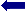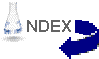A Cooper Pair moving through a lattice.

# Superconductivity Explained

Text courtesy:  Oxford University
Animation courtesy:  Superconductors.ORG and Ian Grant.

Electrical resistance in metals arises because electrons propagating through the solid are scattered due to deviations from perfect translational symmetry. These are produced either by impurities (giving rise to a temperature independent contribution to the resistance) or the phonons - lattice vibrations - in a solid.

In a superconductor below its transition temperature Tc, there is no resistance because these scattering mechanisms are unable to impede the motion of the current carriers. The current is carried in all known classes of superconductor by pairs of electrons known as Cooper pairs. The mechanism by which two negatively charged electrons are bound together is still controversial in "modern" superconducting systems such as the copper oxides or alkali metal fullerides, but well understood in conventional superconductors such as aluminium in terms of the mathematically complex BCS (Bardeen Cooper Schrieffer) theory.

The essential point is that below Tc the binding energy of a pair of electrons causes the opening of a gap in the energy spectrum at Ef (the Fermi energy - the highest occupied level in a solid), separating the pair states from the "normal" single electron states. The size of a Cooper pair is given by the coherence length which is typically 1000Ĺ (though it can be as small as 30Ĺ in the copper oxides). The space occupied by one pair contains many other pairs, and there is thus a complex interdependence of the occupancy of the pair states. There is then insufficient thermal energy to scatter the pairs, as reversing the direction of travel of one electron in the pair requires the destruction of the pair and many other pairs due to the nature of the many-electron BCS wavefunction. The pairs thus carry current unimpeded.

BCS theory applies directly to superconductors such as Nb3Ge (Tc = 23K) in which the electrons are bound together by their interaction with the vibrations of the underlying lattice: one electron in the pair polarizes the lattice by attracting the nuclei towards it, leaving a region of excess positive charge (a potential well) into which a second electron is attracted - the positively charged nuclei thus mediate an attraction between the negatively charged electrons. Only electrons within the vibrational frequency of Ef can be paired by this interaction, and so only a small fraction of the electrons become superconducting.

This is too hard, make it SIMPLER .BACK to "Terminology" page at Superconductors.ORGBACK to "Links" page at Superconductors.ORG